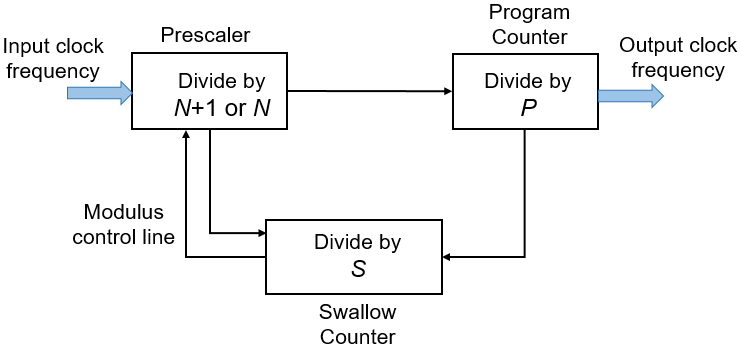Dual Modulus Prescaler

Integer clock divider with two divider ratios

• Library:
• Mixed-Signal Blockset / PLL / Building Blocks

•Description

The Dual Modulus Prescaler subsystem block consists of a program counter, a swallow counter and a prescaler.When the block first receives an input signal, the pulse swallow function is activated. The prescaler divides the input signal frequency by (N+1), where N is defined by the Prescaler divider value (N) parameter. Both the program and swallow counters start counting. The swallow counter resets after counting to S pulses, or (N+1)S cycles, where S is defined by the Swallow counter value (S) parameter. Then, the pulse swallow function is deactivated, and the prescaler divides the input frequency by N.

Since the program counter has already sensed S pulses, it requires (P-S) more pulses, or (P-S)N cycles to reach overflow, where P is defined by the Program counter value (P) parameter. The cycle repeats after both counters are reset.

The effective divider value of the dual modulus prescaler is the ratio of the input frequency to the output frequency:

$\frac{{f}_{\text{in}}}{{f}_{\text{out}}}=\left(N+1\right)S+N\left(P-S\right)=NP+S$

Note

To prevent the program counter and prescaler from resetting prematurely before the swallow counter finishes counting, the condition $P\ge S$ must be met.

The dual modulus prescaler is also known as pulse swallow divider.

Ports

Input

expand all

Input clock frequency, specified as a scalar. In a phase-locked loop (PLL) system, the clk in port is connected to the output port of a VCO block.

Data Types: double

Output

expand all

Output clock frequency, specified as a scalar. In a PLL system, the clk out port is connected to the feedback input port of a PFD block. The output at the clk out port is a square pulse train of 1 V amplitude.

Data Types: double

Parameters

expand all

Maximum value of the program counter, specified as a scalar integer. The counter resets after P cycles.

Programmatic Use

• Use get_param(gcb,'P') to view the current Program counter value value.

• Use set_param(gcb,'P',value) to set Program counter value to a specific value.

Prescaler divider value, specified as a scalar integer. A N/(N+1) dual modulus prescaler divides the input frequency by either N or N+1, depending on the logical state of modulus control line.

Programmatic Use

• Use get_param(gcb,'N') to view the current Prescaler divider value.

• Use set_param(gcb,'N',value) to set Prescaler divider value to a specific value.

Maximum value of the swallow counter, specified as a scalar integer. When the swallow counter resets after S cycles, the pulse swallow function is deactivated.

Programmatic Use

• Use get_param(gcb,'S') to view the current Swallow counter value value.

• Use set_param(gcb,'S',value) to set Swallow counter value to a specific value.

Select to enable increased buffer size during simulation. This increases the buffer size of the Logic Decision inside the Dual Modulus Prescaler block. By default, this option is deselected.

Number of samples of the input buffering available during simulation, specified as a positive integer scalar. This sets the buffer size of the Logic Decision inside the Dual Modulus Prescaler block.

Selecting different simulation solver or sampling strategies can change the number of input samples needed to produce an accurate output sample. Set the Buffer size to a large enough value so that the input buffer contains all the input samples required.

Dependencies

This parameter is only available when Enable increased buffer size option is selected in the Block Parameters dialog box.

Programmatic Use

• Use get_param(gcb,'NBuffer') to view the current value of Buffer size.

• Use set_param(gcb,'NBuffer',value) to set Buffer size to a specific value.